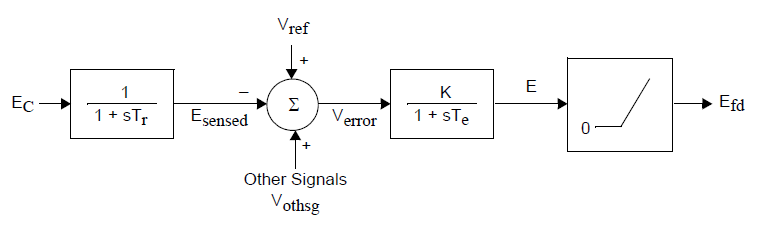# Model Writing in PSS/E

I have just started to learn PSSE model writing. I was looking chapter 23-Model Writing on POM 34.2 Manual, it is an Simple Excitation System, there is one part that I do not understand, what is the meaning between block diagram and code, can someone please tell me? Thank you for your help.

STATE(K)=ECOMP(I)
STATE(K+1)=EFD(I)
VREF(I)=ECOMP(I) + EFD(I)/CON(J+1)

edit retag close merge delete

Sort by » oldest newest most votedThe block diagram for DEMOEX model:The first time constant block has steady state gain 1 and the second block has steady state gain K. The output of time constant blocks are the same as the state of the block. This means that Esensed = Ec and E = Efd at steady state (mode 1). The two states therefore becomes:

STATE(K) = ECOMP(I)
STATE(K+1) = EFD(I)


where ECOMP and EFD are the arrays with measured voltage and field voltage respectively. I is the index for the machine.

The relationship between input and output at steady state is:

(Vref-Ec+Vothsg)*K = Efd

Efd is known (calculated by generator model in mode 1). Ec is terminal voltage if no compensation model is used. Vothsg is zero at steady state. The formula is rewritten to get the necessary value of Vref at initialisation:

Vref = Ec + Efd/K


The initialisation is made backward from the output signal (Efd) and forward from the input signals in mode 1.

more